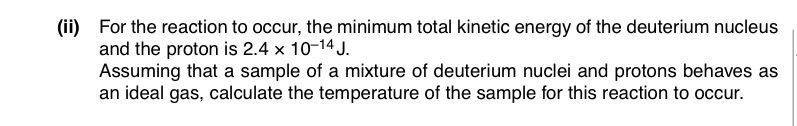# Calculate the temperature for this reaction to occur

• Janiceleong26

#### Janiceleong261. Homework StatementKE = 3/2 R/NA T

## The Attempt at a Solution

By using the formula above, I used 2.4 x 10^-14 J as the mean translational KE , but it should be 1.2x10^-14 , why? I thought the mixture contains both deuterium nucleus and the proton? Why half KE of mixture?

View attachment 100665 1. Homework Statement
View attachment 100666

KE = 3/2 R/NA T

## The Attempt at a Solution

By using the formula above, I used 2.4 x 10^-14 J as the mean translational KE , but it should be 1.2x10^-14 , why? I thought the mixture contains both deuterium nucleus and the proton? Why half KE of mixture?
what happens to the particles kept at some temperature-they get energy and start thermal motion - the average K.E. of particles is related to the temp. and Boltzmann Constant.
3/2 is a factor depending on degree of freedom.
so, what amount of energy a particle say proton will get if you are given with Total K.E. of proton and deuterium? that one should find and then apply the energy-temp relation.

By using the formula above, I used 2.4 x 10^-14 J as the mean translational KE , but it should be 1.2x10^-14 , why? I thought the mixture contains both deuterium nucleus and the proton? Why half KE of mixture?
At a given temperature, how much KE will each particle have? (You have to assume some collisions will be head on.)

what happens to the particles kept at some temperature-they get energy and start thermal motion - the average K.E. of particles is related to the temp. and Boltzmann Constant.
3/2 is a factor depending on degree of freedom.
so, what amount of energy a particle say proton will get if you are given with Total K.E. of proton and deuterium? that one should find and then apply the energy-temp relation.
At a given temperature, how much KE will each particle have? (You have to assume some collisions will be head on.)
Half of the total KE, but why? I thought they are asking for the sample? Why do we need to consider KE of just one of them?

Half of the total KE, but why? I thought they are asking for the sample? Why do we need to consider KE of just one of them?
They are asking for the temperature at which you would expect the reaction to occur. It doesn't have to occur for all particle pairs immediately. Leaving aside that some will have greater than the average KE, they will be moving in various directions. From the temperature you can find the average KE of any given particle, but the reaction involves two particles. Their relative directions of travel matter. Which case makes them most likely to reach the required KE level?

Half of the total KE, but why? I thought they are asking for the sample? Why do we need to consider KE of just one of them?

i think the average velocity of a particle in an enclosure at Temp. T may be taken as v(rms) and
1/2 . m(i). v(i)^2 = 3/2 k T ; now one has to check how the total KE will be shared.

now one has to check how the total KE will be shared.
Not sure what you mean by that. Shared between what?

Shared between what?

shared between the two kinds of particles namely deuteron and protons.

shared between the two kinds of particles namely deuteron and protons.

well it does -but the question is being asked as to why half of the total KE of the two (given in the question) is being used for estimating T.

They are asking for the temperature at which you would expect the reaction to occur. It doesn't have to occur for all particle pairs immediately. Leaving aside that some will have greater than the average KE, they will be moving in various directions. From the temperature you can find the average KE of any given particle, but the reaction involves two particles. Their relative directions of travel matter. Which case makes them most likely to reach the required KE level?
•